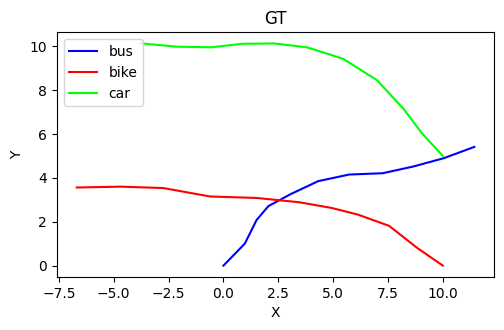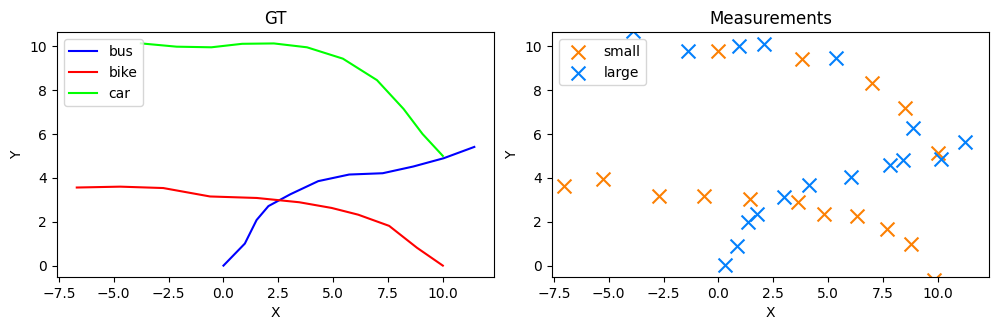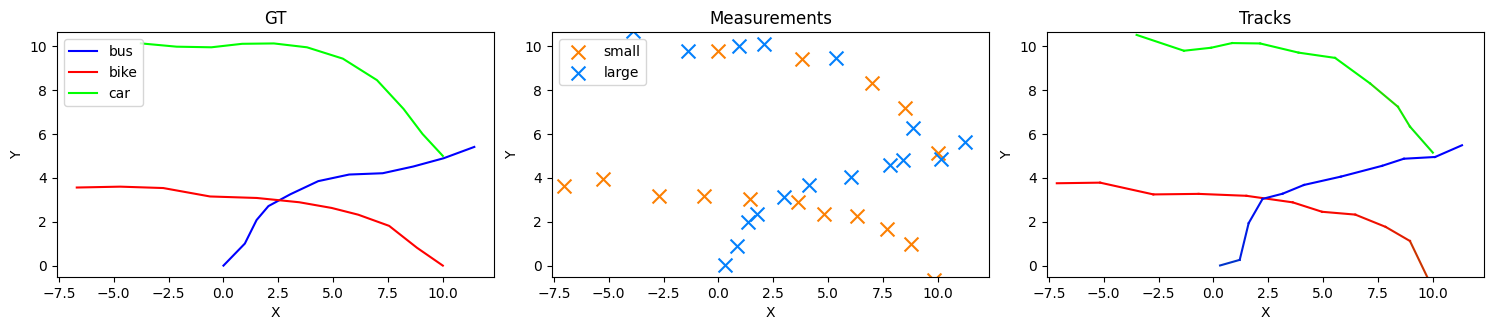# Disjoint Tracking and Classification

This is a demonstration of a utilisation of the implemented Hidden Markov model and composite tracking modules in order to categorise a target as well as track its kinematics.

All non-generic imports will be given in order of usage.

```from datetime import datetime, timedelta

import matplotlib.pyplot as plt
import numpy as np

from stonesoup.models.transition.linear import ConstantVelocity, \
CombinedLinearGaussianTransitionModel
from stonesoup.types.groundtruth import GroundTruthState
```

## Ground Truth, Categorical and Composite States

We will attempt to track and classify 3 targets.

### True Kinematics

They will move in random directions from defined starting points.

```start = datetime.now()

kinematic_state1 = GroundTruthState([0, 1, 0, 1], timestamp=start)  # x, vx, y, vy
kinematic_state2 = GroundTruthState([10, -1, 0, 1], timestamp=start)
kinematic_state3 = GroundTruthState([10, -1, 5, 1], timestamp=start)

kinematic_transition = CombinedLinearGaussianTransitionModel([ConstantVelocity(0.1),
ConstantVelocity(0.1)])
```

### True Classifications

A target may take one of three discrete hidden classes: ‘bike’, ‘car’ and ‘bus’. It will be assumed that the targets cannot transition from one class to another, hence an identity transition matrix is given to the `MarkovianTransitionModel` for all targets.

A `CategoricalState` class is used to store information on the classification/’category’ of the targets. The state vector of each will define a categorical distribution over the 3 possible classes, whereby each component defines the probability that the target is of the corresponding class. For example, the state vector (0.2, 0.3, 0.5), with category names (‘bike’, ‘car’, ‘bus’) indicates that the target has a 20% probability of being class ‘bike’, a 30% probability of being class ‘car’ etc. It does not make sense to have a true target being a distribution over the possible classes, and therefore the true categorical states will have binary state vectors indicating a specific class for each target (i.e. a ‘1’ at one state vector index, and ‘0’s elsewhere). The `CategoricalGroundTruthState` inherits directly from the base `CategoricalState`.

```from stonesoup.types.groundtruth import CategoricalGroundTruthState
from stonesoup.models.transition.categorical import MarkovianTransitionModel

hidden_classes = ['bike', 'car', 'bus']
gt_kwargs = {'timestamp': start, 'categories': hidden_classes}
category_state1 = CategoricalGroundTruthState([0, 0, 1], **gt_kwargs)
category_state2 = CategoricalGroundTruthState([1, 0, 0], **gt_kwargs)
category_state3 = CategoricalGroundTruthState([0, 1, 0], **gt_kwargs)

category_transition = MarkovianTransitionModel(transition_matrix=np.eye(3))
```

### Composite States

Each target will have kinematics and a category to be inferred. These are contained within a `CompositeState` type (in this instance the child class `CompositeGroundTruthState`).

```from stonesoup.types.groundtruth import CompositeGroundTruthState

initial_state1 = CompositeGroundTruthState([kinematic_state1, category_state1])
initial_state2 = CompositeGroundTruthState([kinematic_state2, category_state2])
initial_state3 = CompositeGroundTruthState([kinematic_state3, category_state3])
```

### Generating Ground Truth Paths

Both the physical and categorical states of the targets need to be transitioned. While the category will remain the same, a transition model is used here for the sake of demonstration.

```from stonesoup.types.groundtruth import GroundTruthPath

GT1 = GroundTruthPath([initial_state1], id='GT1')
GT2 = GroundTruthPath([initial_state2], id='GT2')
GT3 = GroundTruthPath([initial_state3], id='GT3')
ground_truth_paths = [GT1, GT2, GT3]

for GT in ground_truth_paths:
for i in range(10):
kinematic_sv = kinematic_transition.function(GT[-1],
noise=True,
time_interval=timedelta(seconds=1))
kinematic = GroundTruthState(kinematic_sv,
timestamp=GT[-1].timestamp + timedelta(seconds=1))

category_sv = category_transition.function(GT[-1],
noise=True,
time_interval=timedelta(seconds=1))
category = CategoricalGroundTruthState(category_sv,
timestamp=GT[-1].timestamp + timedelta(seconds=1),
categories=hidden_classes)

GT.append(CompositeGroundTruthState([kinematic, category]))

# Printing GT1
for state in GT1:
vector = np.round(state.state_vector.flatten().astype(np.double), 2)
print("%25s" % vector, ' -- ', state.category, ' -- ', state.timestamp)
```
```            [0. 1. 0. 1.]  --  bus  --  2023-05-30 10:32:25.425901
[0.97 0.83 1.   1.19]  --  bus  --  2023-05-30 10:32:26.425901
[1.51 0.53 2.08 1.06]  --  bus  --  2023-05-30 10:32:27.425901
[2.05 0.66 2.71 0.52]  --  bus  --  2023-05-30 10:32:28.425901
[3.05 1.37 3.25 0.64]  --  bus  --  2023-05-30 10:32:29.425901
[4.32 1.31 3.85 0.5 ]  --  bus  --  2023-05-30 10:32:30.425901
[5.73 1.38 4.15 0.19]  --  bus  --  2023-05-30 10:32:31.425901
[7.26 1.52 4.21 0.16]  --  bus  --  2023-05-30 10:32:32.425901
[8.65 1.52 4.52 0.33]  --  bus  --  2023-05-30 10:32:33.425901
[10.04  1.49  4.89  0.58]  --  bus  --  2023-05-30 10:32:34.425901
[11.43  1.32  5.41  0.58]  --  bus  --  2023-05-30 10:32:35.425901
```

### Plotting Ground Truths

Colour will be used in plotting as an indicator to category: red == ‘bike’, green == ‘car’, blue == ‘bus’.

```fig, axes = plt.subplots(1, 3, figsize=(10, 5))
fig.set_figheight(15)
fig.set_figwidth(15)
fig.tight_layout()

for ax in axes:
ax.set_aspect('equal', 'box')

for GT in ground_truth_paths:
X = list()
Y = list()
col = list(GT.state_vector)
for state in GT:
pos = state.state_vector
X.append(pos)
Y.append(pos)
axes.plot(X, Y, color=col, label=GT[-1].category)
axes.legend(loc='upper left')
axes.set(title='GT', xlabel='X', ylabel='Y')
axes.set_visible(False)
axes.set_visible(False)

def set_axes_limits():
xmax = max(ax.get_xlim() for ax in axes)
ymax = max(ax.get_ylim() for ax in axes)
xmin = min(ax.get_xlim() for ax in axes)
ymin = min(ax.get_ylim() for ax in axes)
for ax in axes:
ax.set_xlim(xmin, xmax)
ax.set_ylim(ymin, ymax)

set_axes_limits()
```## Measurement

A new sensor will be created, that can provide the information needed to both track and classify the targets.

### Composite Detection

Detections relating to both the kinematics and classification will be needed. Therefore we will create a sensor that outputs `CompositeDetection` types. The input sensors list will provide the contents of these compositions. For this example we will provide a `RadarBearingRange` and a `HMMSensor` for kinematics and classification respectively. `CompositeDetection` types have a mapping attribute, which defines what sub-state index each sub-detection was created from. For example, with a composite state of form: (kinematic state, categorical state), and composite detection with mapping (1, 0), this would indicate that the 0th index sub-detection was attained from the categorical state, and the 1st index sub-detection from the kinematic state.

```from typing import Set, Union, Sequence

from stonesoup.base import Property
from stonesoup.sensor.sensor import Sensor
from stonesoup.types.detection import CompositeDetection

class CompositeSensor(Sensor):
sensors: Sequence[Sensor] = Property(doc="A list of sensors.")
mapping: Sequence = Property(default=None,
doc="Mapping of which component states in the composite truth "
"state is measured.")

def __init__(self, *args, **kwargs):
super().__init__(*args, **kwargs)
if self.mapping is None:
self.mapping = list(np.arange(len(self.sensors)))

def measure(self, ground_truths: Set[CompositeGroundTruthState],
noise: Sequence[Union[np.ndarray, bool]] = True,
**kwargs) -> Set[CompositeDetection]:

if isinstance(noise, bool) or len(noise) == 1:
noise = len(self.sensors) * [noise]

detections = set()
for truth in ground_truths:

sub_detections = list()

states = [truth.sub_states[i] for i in self.mapping]

for state, sub_sensor, sub_noise in zip(states, self.sensors, noise):
sub_detection = sub_sensor.measure(
ground_truths={state},
noise=sub_noise
).pop()  # sub-sensor returns a set
sub_detections.append(sub_detection)

detection = CompositeDetection(sub_states=sub_detections,
groundtruth_path=truth,
mapping=self.mapping)

return detections

@property
def measurement_model(self):
raise NotImplementedError
```

### Kinematic Measurement

Measurements of the target’s kinematics will be attained via a `RadarBearingRange` sensor model.

```from stonesoup.sensor.radar.radar import RadarBearingRange

position_mapping=[0, 2],
```

### Categorical Measurement

Using the hidden Markov model, it is assumed the hidden class of the target cannot be directly observed, and instead indirect observations are taken. In this instance, observations of the target’s size are taken (‘small’ or ‘large’), which have direct implications as to the target’s hidden class, and this relationship is modelled by the emission matrix of the `MarkovianMeasurementModel`, which is used by the `HMMSensor` to provide `CategoricalDetection` types. We will model this such that a ‘bike’ has a very small chance of being observed as a ‘big’ target. Similarly, a ‘bus’ will tend to appear as ‘large’. Whereas, a ‘car’ has equal chance of being observed as either.

```from stonesoup.models.measurement.categorical import MarkovianMeasurementModel
from stonesoup.sensor.categorical import HMMSensor

E = np.array([[0.99, 0.5, 0.01],  # P(small | bike), P(small | car), P(small | bus
[0.01, 0.5, 0.99]])
model = MarkovianMeasurementModel(emission_matrix=E,
measurement_categories=['small', 'large'])

eo = HMMSensor(measurement_model=model)
```

### Composite Sensor

Creating the composite sensor class.

```sensor = CompositeSensor(sensors=[eo, radar], mapping=[1, 0])
```

### Generating Measurements

```all_measurements = list()

for gts1, gts2, gts3 in zip(GT1, GT2, GT3):
measurements_at_time = sensor.measure({gts1, gts2, gts3})
timestamp = gts1.timestamp
all_measurements.append((timestamp, measurements_at_time))

# Printing some measurements
for i, (time, measurements_at_time) in enumerate(all_measurements):
if i > 2:
break
print(f"{time:%H:%M:%S}")
for measurement in measurements_at_time:
vector = np.round(measurement.state_vector.flatten().astype(np.double), 2)
print("%25s" % vector, ' -- ', measurement.category)
```
```10:32:25
[ 1.    0.    0.48 11.25]  --  small
[0.   1.   0.02 0.3 ]  --  large
[ 1.    0.   -0.07  9.83]  --  small
10:32:26
[0.   1.   0.8  1.21]  --  large
[1.   0.   0.11 8.85]  --  small
[ 0.    1.    0.61 10.86]  --  large
10:32:27
[ 1.    0.    0.7  11.12]  --  small
[0.   1.   0.98 2.41]  --  large
[1.   0.   0.21 7.86]  --  small
```

### Plotting Measurements

Colour will be used to indicate measurement category: orange == ‘small’, light-blue == ‘large’. It is expected that the bus will have mostly light-blue (large) measurements coinciding with its route, the bike will have mostly orange (small), and the car a roughly even split of both.

```for time, measurements in all_measurements:
for measurement in measurements:
loc = measurement.state_vector
obs = measurement.state_vector
col = list(measurement.measurement_model.emission_matrix.T @ obs)

phi = loc
rho = loc
x = rho * np.cos(phi)
y = rho * np.sin(phi)
axes.scatter(x, y, color=col, marker='x', s=100, label=measurement.category)

a = axes.get_legend_handles_labels()
b = {l: h for h, l in zip(*a)}
c = [*zip(*b.items())]
d = c[::-1]
axes.legend(*d, loc='upper left')

axes.set(title='Measurements', xlabel='X', ylabel='Y')
axes.set_visible(True)
set_axes_limits()
fig
```## Tracking Components

### Predictor

Though not used by the tracking components here, a `CompositePredictor` will predict the component states of a composite state forward, according to a list of sub-predictors.

A `HMMPredictor` specifically uses `MarkovianTransitionModel` types to predict.

```from stonesoup.predictor.kalman import KalmanPredictor
from stonesoup.predictor.categorical import HMMPredictor
from stonesoup.predictor.composite import CompositePredictor

kinematic_predictor = KalmanPredictor(kinematic_transition)
category_predictor = HMMPredictor(category_transition)

predictor = CompositePredictor([kinematic_predictor, category_predictor])
```

### Updater

The `CompositeUpdater` composite updater will update each component sub-state according to a list of corresponding sub-updaters. It has no method to create measurement predictions. This is instead handled on instantiation of `CompositeHypothesis` types: the expected arguments to the updater’s update method.

```from stonesoup.updater.kalman import ExtendedKalmanUpdater
from stonesoup.updater.categorical import HMMUpdater
from stonesoup.updater.composite import CompositeUpdater

kinematic_updater = ExtendedKalmanUpdater()
category_updater = HMMUpdater()

updater = CompositeUpdater(sub_updaters=[kinematic_updater, category_updater])
```

### Hypothesiser

The hypothesiser is a `CompositeHypothesiser` type. It is in the data association step that tracking and classification are combined: for each measurement, a hypothesis is created for both a track’s kinematic and categorical components. A `CompositeHypothesis` type is created, which contains these sub-hypotheses, whereby its weight is equal to the product of the sub-hypotheses’ weights. These sub-hypotheses should be probabilistic.

The `CompositeHypothesiser` uses a list of sub-hypothesisers to create these sub-hypotheses, hence the sub-hypothesisers should also be probabilistic. In this example we will define a hypothesiser that simply changes kinematic distance weights in to probabilities for hypothesising the kinematic sub-state of the track.

```from stonesoup.measures import Mahalanobis
from stonesoup.hypothesiser.distance import DistanceHypothesiser
from stonesoup.types.hypothesis import SingleProbabilityHypothesis
from stonesoup.types.multihypothesis import MultipleHypothesis

class ProbabilityHypothesiser(DistanceHypothesiser):
def hypothesise(self, track, detections, timestamp, **kwargs):
multi_hypothesis = super().hypothesise(track, detections, timestamp, **kwargs)
single_hypotheses = multi_hypothesis.single_hypotheses
prob_single_hypotheses = list()
for hypothesis in single_hypotheses:
prob_hypothesis = SingleProbabilityHypothesis(hypothesis.prediction,
hypothesis.measurement,
1 / hypothesis.distance,
hypothesis.measurement_prediction)
prob_single_hypotheses.append(prob_hypothesis)
return MultipleHypothesis(prob_single_hypotheses, normalise=False, total_weight=1)

kinematic_hypothesiser = ProbabilityHypothesiser(predictor=kinematic_predictor,
updater=kinematic_updater,
measure=Mahalanobis())
```

A `HMMHypothesiser` is used for calculating categorical hypotheses. It utilises the `ObservationAccuracy` measure: a multi-dimensional extension of an ‘accuracy’ score, essentially providing a measure of the similarity between two categorical distributions.

```from stonesoup.hypothesiser.categorical import HMMHypothesiser
from stonesoup.hypothesiser.composite import CompositeHypothesiser

category_hypothesiser = HMMHypothesiser(predictor=category_predictor,
updater=category_updater)
hypothesiser = CompositeHypothesiser(
sub_hypothesisers=[kinematic_hypothesiser, category_hypothesiser]
)
```

### Data Associator

We will use a standard `GNNWith2DAssignment` data associator.

```from stonesoup.dataassociator.neighbour import GNNWith2DAssignment

data_associator = GNNWith2DAssignment(hypothesiser)
```

### Prior

As we are tracking in a composite state space, we should initiate tracks with a `CompositeState` type. The kinematic sub-state of the prior is a usual Gaussian state. For the categorical sub-state of the prior, equal probability is given to all 3 of the possible hidden classes that a target might take (the category names are also provided here).

```from stonesoup.types.state import GaussianState, CategoricalState, CompositeState

kinematic_prior = GaussianState([0, 0, 0, 0], np.diag([10, 10, 10, 10]))
category_prior = CategoricalState([1 / 3, 1 / 3, 1 / 3], categories=hidden_classes)
prior = CompositeState([kinematic_prior, category_prior])
```

### Initiator

The initiator is composite. For each unassociated detection, a new track will be initiated. In this instance we use a `CompositeUpdateInitiator` type. A detection has both kinematic and categorical information to initiate the 2 state space sub-states from. However, in an instance where a detection only provides one of these, the missing sub-state for the track will be initiated as the given prior’s sub-state (eg. if a detection provides only kinematic information of the target, the track will initiate its categorical sub-state as the category_prior defined earlier).

```from stonesoup.initiator.simple import SimpleMeasurementInitiator
from stonesoup.initiator.categorical import SimpleCategoricalMeasurementInitiator
from stonesoup.initiator.composite import CompositeUpdateInitiator

kinematic_initiator = SimpleMeasurementInitiator(prior_state=kinematic_prior,
measurement_model=None)
category_initiator = SimpleCategoricalMeasurementInitiator(prior_state=category_prior,
updater=category_updater)
initiator = CompositeUpdateInitiator(sub_initiators=[kinematic_initiator, category_initiator])
```

### Deleter

We can use a standard `UpdateTimeStepsDeleter`.

```from stonesoup.deleter.time import UpdateTimeStepsDeleter

deleter = UpdateTimeStepsDeleter(2)
```

### Tracker

We can use a standard `MultiTargetTracker`.

```from stonesoup.tracker.simple import MultiTargetTracker

tracker = MultiTargetTracker(initiator, deleter, all_measurements, data_associator, updater)
```

## Tracking

```tracks = set()
for time, ctracks in tracker:
tracks.update(ctracks)

print(f'Number of tracks {len(tracks)}')
for track in tracks:
print(f'id: {track.id}')
for state in track:
vector = np.round(state.state_vector.flatten().astype(np.double), 2)
print("%25s" % vector, ' -- ', state.category, ' -- ', state.timestamp)
```
```Number of tracks 3
id: 07a292b0-1d4c-4794-bb25-988d078b8aa4
[ 9.81  0.   -0.66  0.  ]  --  bike  --  2023-05-30 10:32:25.425901
[ 8.96 -0.85  1.12  1.77]  --  bike  --  2023-05-30 10:32:26.425901
[ 7.85 -1.02  1.76  0.99]  --  bike  --  2023-05-30 10:32:27.425901
[ 6.46 -1.25  2.33  0.7 ]  --  bike  --  2023-05-30 10:32:28.425901
[ 4.95 -1.4   2.45  0.28]  --  bike  --  2023-05-30 10:32:29.425901
[ 3.61 -1.37  2.88  0.39]  --  bike  --  2023-05-30 10:32:30.425901
[ 1.5  -1.91  3.18  0.41]  --  bike  --  2023-05-30 10:32:31.425901
[-0.68 -2.1   3.27  0.2 ]  --  bike  --  2023-05-30 10:32:32.425901
[-2.74 -2.07  3.25  0.05]  --  bike  --  2023-05-30 10:32:33.425901
[-5.17 -2.3   3.78  0.37]  --  bike  --  2023-05-30 10:32:34.425901
[-7.14 -2.1   3.75  0.09]  --  bike  --  2023-05-30 10:32:35.425901
id: 441029df-794b-4e05-9c87-78a2b03aba49
[0.3  0.   0.01 0.  ]  --  bus  --  2023-05-30 10:32:25.425901
[1.2  0.89 0.26 0.26]  --  bus  --  2023-05-30 10:32:26.425901
[1.6  0.55 1.94 2.03]  --  bus  --  2023-05-30 10:32:27.425901
[2.24 0.77 3.04 1.05]  --  bus  --  2023-05-30 10:32:28.425901
[3.15 0.99 3.28 0.45]  --  bus  --  2023-05-30 10:32:29.425901
[4.12 0.98 3.68 0.42]  --  bus  --  2023-05-30 10:32:30.425901
[5.81 1.5  4.06 0.32]  --  bus  --  2023-05-30 10:32:31.425901
[7.69 1.75 4.55 0.42]  --  bus  --  2023-05-30 10:32:32.425901
[8.68 1.25 4.87 0.38]  --  bus  --  2023-05-30 10:32:33.425901
[10.1   1.36  4.95  0.18]  --  bus  --  2023-05-30 10:32:34.425901
[11.33  1.28  5.49  0.41]  --  bus  --  2023-05-30 10:32:35.425901
id: b41e7acb-b4e7-41b4-bef6-1f9572fc74a4
[10.    0.    5.15  0.  ]  --  bike  --  2023-05-30 10:32:25.425901
[ 8.96 -1.03  6.34  1.18]  --  car  --  2023-05-30 10:32:26.425901
[ 8.41 -0.71  7.24  1.  ]  --  car  --  2023-05-30 10:32:27.425901
[ 7.14 -1.06  8.3   1.04]  --  car  --  2023-05-30 10:32:28.425901
[ 5.54 -1.4   9.47  1.11]  --  car  --  2023-05-30 10:32:29.425901
[ 3.88 -1.58  9.71  0.55]  --  car  --  2023-05-30 10:32:30.425901
[ 2.13 -1.69 10.13  0.46]  --  car  --  2023-05-30 10:32:31.425901
[ 0.85 -1.42 10.14  0.17]  --  car  --  2023-05-30 10:32:32.425901
[-0.11 -1.12  9.93 -0.08]  --  car  --  2023-05-30 10:32:33.425901
[-1.35 -1.2   9.79 -0.12]  --  car  --  2023-05-30 10:32:34.425901
[-3.5  -1.84 10.52  0.43]  --  car  --  2023-05-30 10:32:35.425901
```

### Plotting Tracks

Colour will be used to indicate a track’s hidden category distribution. The rgb value is defined by the ‘bike’, ‘car’, and ‘bus’ probabilities. For example, a track with high probability of being a ‘bike’ will have a high ‘r’ value, and hence appear more red.

```for track in tracks:
for i, state in enumerate(track[1:], 1):
loc0 = track[i - 1].state_vector.flatten()
loc1 = state.state_vector.flatten()
X = [loc0, loc1]
Y = [loc0, loc1]
axes.plot(X, Y, label='track', color=list(state.state_vector))

axes.set(title='Tracks', xlabel='X', ylabel='Y')
axes.set_visible(True)
set_axes_limits()
fig
```Total running time of the script: ( 0 minutes 1.431 seconds)

Gallery generated by Sphinx-Gallery